2019-01-21 22:21:54 qq_42887760 阅读数 850
• ###### MATLAB图像处理

全面系统的学习MATLAB在图像处理中的应用

19926 人正在学习 去看看 魏伟

# 矩的概念介绍

• 几何矩( 其中P(i,j)为图像在坐标点(i,j)处的灰度值。)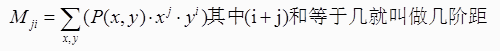• 中心距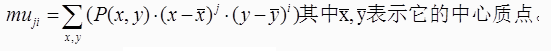• 中心归一化距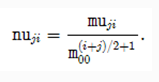其中：图像中心Center(x0, y0)。 此非图像坐标的中心点，而是图像 质 的中心，质点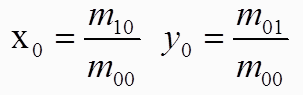# API介绍与使用 – cv::moments 计算生成数据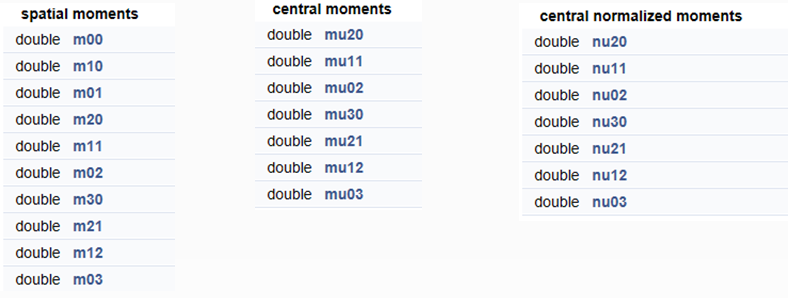# API介绍与使用-计算矩cv::moments

opencv中提供了moments()来计算图像中的中心矩(最高到三阶)，HuMoments()用于由中心矩计算Hu矩.同时配合函数contourArea函数计算轮廓面积和arcLength来计算轮廓或曲线长度

• moments( // 计算矩，返回 0阶到3阶所有的几何矩，2阶到3阶所有的中心矩，2阶到3阶所有的中心归一矩 InputArray array,//输入数据，findContours 找到的轮廓数据 bool binaryImage=false // 是否为二值图像 )

1. array:输入数组，可以是光栅图像(单通道，8-bit或浮点型二维数组),或者是一个二维数组(1 X N或N X 1),二维数组类型为Point或Point2f
2. binaryImage:默认值是false，如果为true，则所有非零的像素都会按值1对待，也就是说相当于对图像进行了二值化处理，阈值为1，此参数仅对图像有效。
• contourArea( InputArray contour,//输入轮廓数据 bool oriented// 默认false、返回绝对值)

1. contour:是一个向量，二维点，可以是vector或Mat类型
2. oriented:有默认值false，面向区域标识符，如果为true，该函数返回一个带符号的面积，其正负取决于轮廓的方向(顺时针还是逆时针)。根据这个特性可以根据面积的符号来确定轮廓的位置。如果是默认值false，则面积以绝对值的形式返回.
3. 该函数使用Green formula计算轮廓面积，返回面积和非零像素数量如果使用drawContours或fillPoly绘制轮廓，可能导致不同。
• arcLength( //用于计算封闭轮廓的周长或曲线的长度 InputArray curve,//输入曲线数据 bool closed// 是否是封闭曲线)

1. curve:输入二维点集，可以是vector或Mat类型
2. closed:曲线是否封闭的标志位，true则封闭否则不封闭

# 演示代码-步骤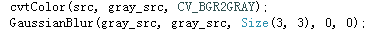1. 提取图像边缘
2. 发现轮廓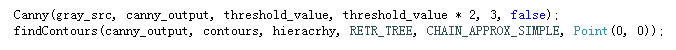3. 计算每个轮廓对象的矩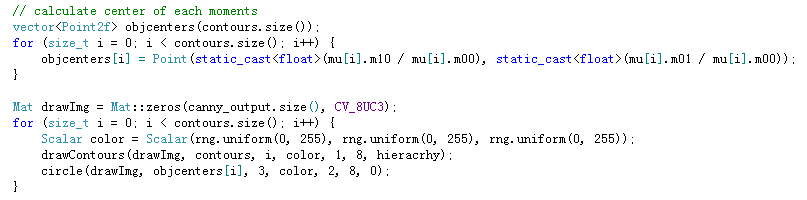4. 计算每个对象的中心、弧长、面积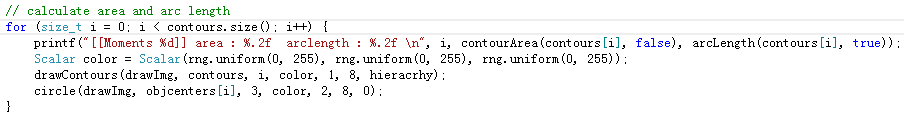# 程序代码

#include <opencv2/opencv.hpp>
#include <iostream>
using namespace cv;
using namespace std;

//定义全局变量
Mat src; Mat src_gray;
int thresh = 100;
int max_thresh = 255;
RNG rng(12345);
//声明回调函数
void thresh_callback(int, void* );

int main( int argc, char** argv )
{
// 读入原图像, 返回3通道图像数据
// 创建新窗口
char* source_window = "Source";
namedWindow( source_window, CV_WINDOW_AUTOSIZE );
imshow( source_window, src );

// 把原图像转化成灰度图像并进行平滑
cvtColor( src, src_gray, CV_BGR2GRAY );
//blur( src_gray, src_gray, Size(3,3) );
GaussianBlur(src_gray, src_gray, Size(3, 3), 0, 0);//这里用高斯模糊去噪，相比于均值，轮廓发现的准确
imshow("灰度图", src_gray);

createTrackbar( " Canny thresh:", "Source", &thresh, max_thresh, thresh_callback );
thresh_callback( thresh, 0 );

waitKey(0);
return(0);
}

void thresh_callback(int, void* )
{
Mat canny_output;
vector<vector<Point> > contours;
vector<Vec4i> hierarchy;

// 使用Canndy检测边缘
Canny( src_gray, canny_output, thresh, thresh*2, 3 );//提取边缘
// 找到轮廓
findContours( canny_output, contours, hierarchy, CV_RETR_TREE, CV_CHAIN_APPROX_SIMPLE, Point(0, 0) );//发现轮廓

// 计算图像矩
vector<Moments> mu(contours.size() );//存放各阶各种矩
for( int i = 0; i < contours.size(); i++ ){
//计算矩，返回 0阶到3阶所有的几何矩，2阶到3阶所有的中心矩，2阶到3阶所有的中心归一矩
mu[i] = moments( contours[i], false );
}

//  计算中心矩，即图像的质心：
vector<Point2f> mc( contours.size() );//各阶各种矩的图像中心，质点
for( int i = 0; i < contours.size(); i++ ){
mc[i] = Point2f( mu[i].m10/mu[i].m00 , mu[i].m01/mu[i].m00 );
}

// 绘制轮廓
Mat drawing = Mat::zeros( canny_output.size(), CV_8UC3 );
for( int i = 0; i< contours.size(); i++ ){
Scalar color = Scalar( rng.uniform(0, 255), rng.uniform(0,255), rng.uniform(0,255) );
drawContours( drawing, contours, i, color, 2, 8, hierarchy, 0, Point() );//绘制轮廓
circle( drawing, mc[i], 4, color, -1, 8, 0 );//绘制质点
}

// 显示到窗口中
namedWindow( "Contours", CV_WINDOW_AUTOSIZE );
imshow( "Contours", drawing );

// 通过m00计算轮廓面积并且和OpenCV函数比较
printf("\t Info: Area and Contour Length \n");
for( int i = 0; i< contours.size(); i++ ){
printf(" * Contour[%d] - Area (M_00) = %.2f - Area OpenCV: %.2f - Length: %.2f \n", i, mu[i].m00, contourArea(contours[i]), arcLength( contours[i], true ) );
Scalar color = Scalar( rng.uniform(0, 255), rng.uniform(0,255), rng.uniform(0,255) );
drawContours( drawing, contours, i, color, 2, 8, hierarchy, 0, Point() );
circle( drawing, mc[i], 4, color, -1, 8, 0 );
}
}



# 运行截图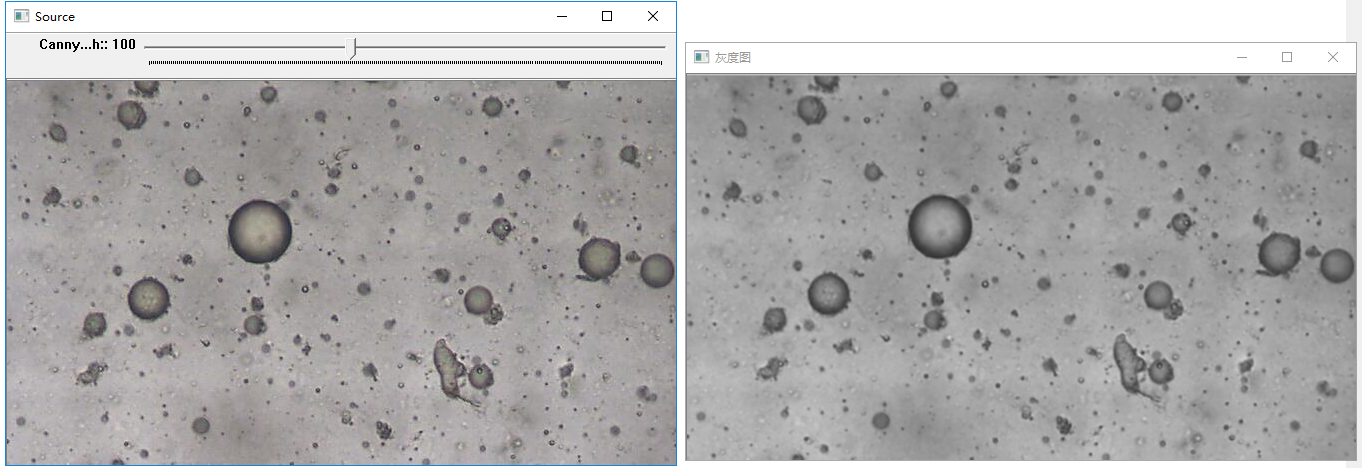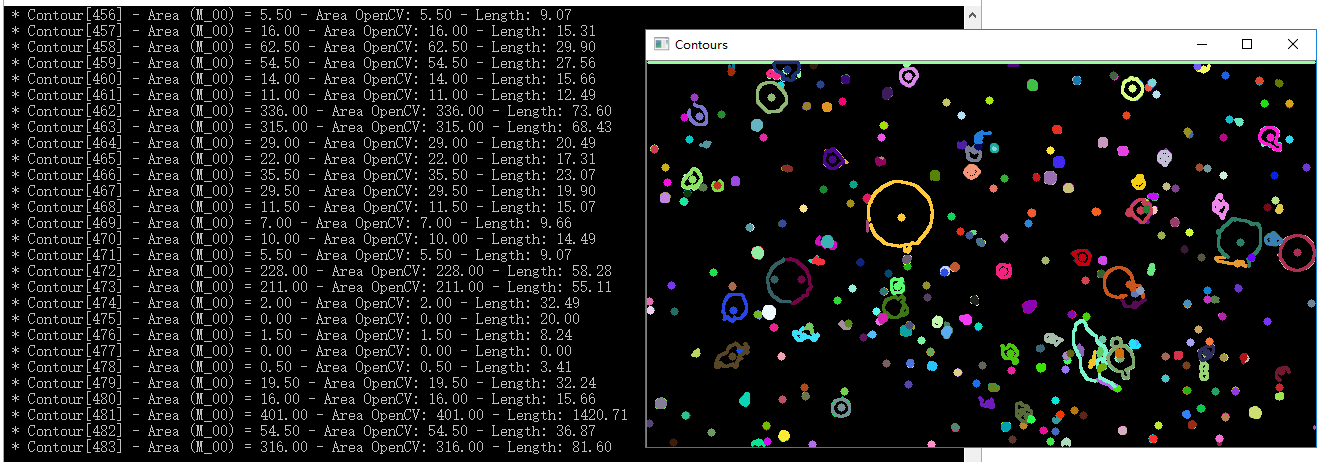### 参考博客

2016-08-01 12:52:15 u013496193 阅读数 374
• ###### MATLAB图像处理

全面系统的学习MATLAB在图像处理中的应用

19926 人正在学习 去看看 魏伟

## 距离度量

（1）D(p,q)>=0;
（2）D(p,q)=D(q,p)
（3）D（p,z)<=D(p,q)+D(q,z);

1.欧式距离

2.城市街区距离

3.棋盘距离

2019-04-14 09:09:41 mary_0830 阅读数 190
• ###### MATLAB图像处理

全面系统的学习MATLAB在图像处理中的应用

19926 人正在学习 去看看 魏伟

# （一）在频域中直接生成滤波器

## （1）建立网格数组以实现频域滤波器

function [U,V] = dftuv(M,N)   % dftuv的M-函数
u = single(0:(M-1));
v = single(0:(N-1));    %建立变量范围
idx = find(u >M/2);
u(idx) = u(idx) - M;
idy = find(v >N/2);
v(idy) = v(idy) - N;  %计算在meshgrid中使用的索引
[V,U] = meshgrid(v,u);   %计算在meshgrid中行列


[U,V]=dftuv(8,5);
DSQ= U.^2+V.^2
fftshift(DSQ)    %使用函数 fftshift 得到频域矩形中心的距离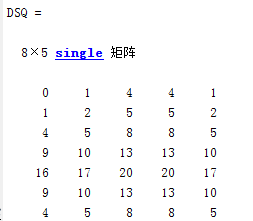fftshift(DSQ) 结果，如图：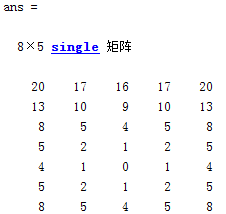## （2）频域低通（平滑）滤波器

f = imread('C:\Users\Public\Pictures\Sample Pictures\Fig0313(a).tif ');
[f,revertclass] = tofloat(f);
[U,V] = dftuv(PQ(1),PQ(2));
D = hypot(U,V);
D0 = 0.05*PQ(2);
F = fft2(f,PQ(1),PQ(2));
H = exp(-(D.^2)/(2*(D0^2)));
g = dftfilt(f,H);
g = revertclass(g);
subplot(221),imshow(f), title('原图像');
subplot(222),imshow(fftshift(H)), title('以图像显示的高斯低通滤波器');
subplot(223),imshow(log(1+abs(fftshift(F))),[]), title('滤波后的图像谱');
subplot(224),imshow(g), title('滤波后的图像谱');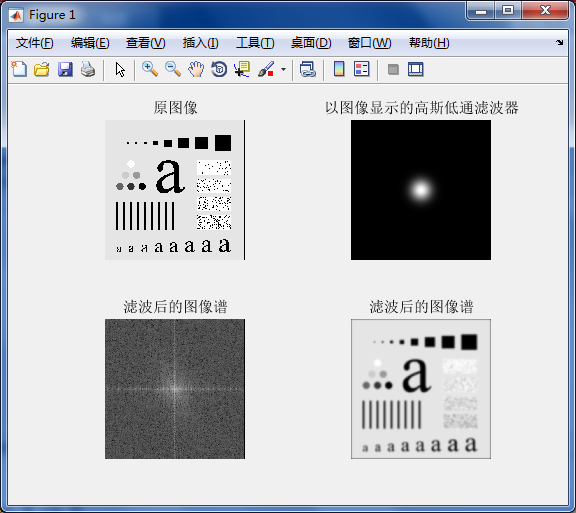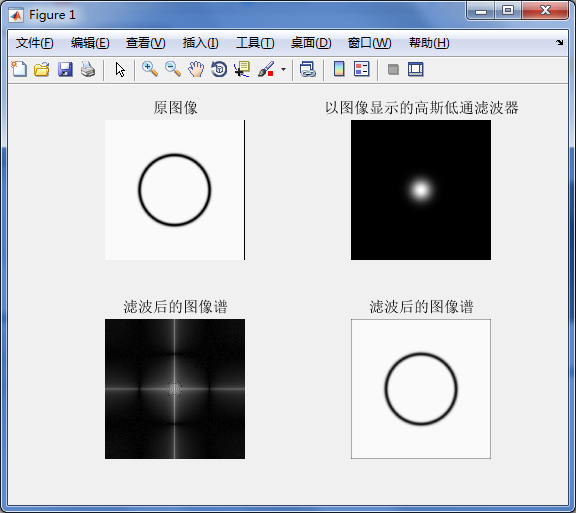## （3）线框及表面绘制

mesh(H)
mesh(H(1:k:end,1:k:end))       %用于绘制第k个点
colormap([0 0 0])            %用于设置线框颜色（[0 0 0]黑色，默认值是彩色线框）
gird off                    %关掉网格命令
axis off            %关掉轴的命令
view(az,el)     %设置观察者位置


H = fftshift(lpfilter('gaussian',500,500,50));
mesh(double(H(1:10:500,1:10:500)))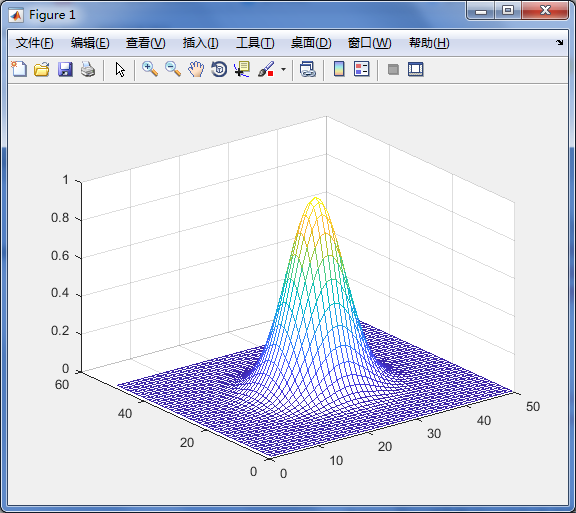H = fftshift(lpfilter('gaussian',500,500,50));
mesh(double(H(1:10:500,1:10:500)))    %建立线框图
axis tight           % 产生线框图
colormap([0 0 0])     %将线框颜色变成黑色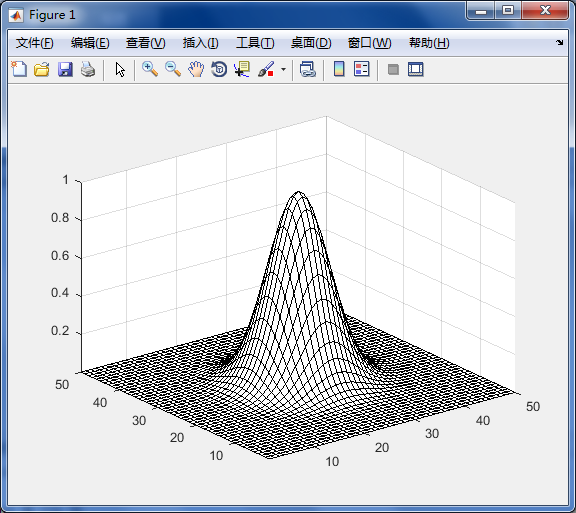colormap([0 1 0])     %将线框颜色变成绿色，三个数字分别表示红绿蓝，且colormap的值必须位于【0,1】之间。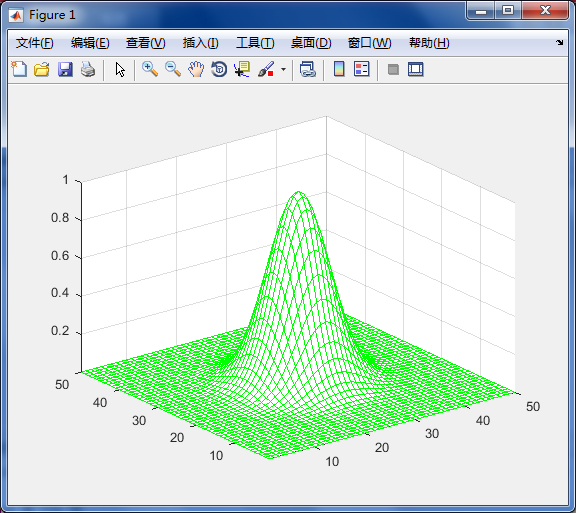colormap([0.8 1 0])     %将线框颜色变成淡黄色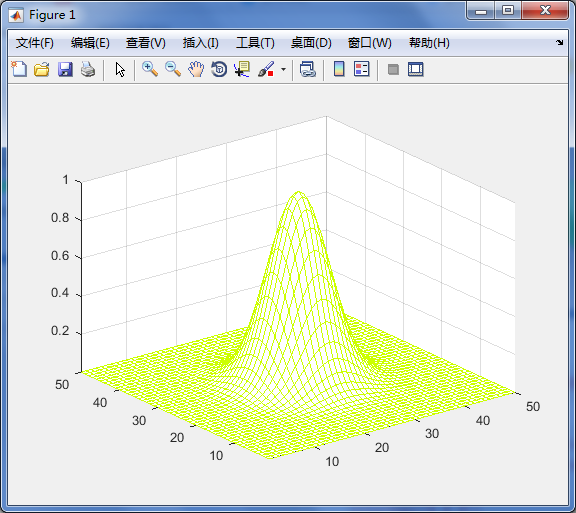H = fftshift(lpfilter('gaussian',500,500,50));
mesh(double(H(1:10:500,1:10:500)))
axis tight
colormap([0 0 0])
axis off         %消除坐标轴
grid off        %消除栅格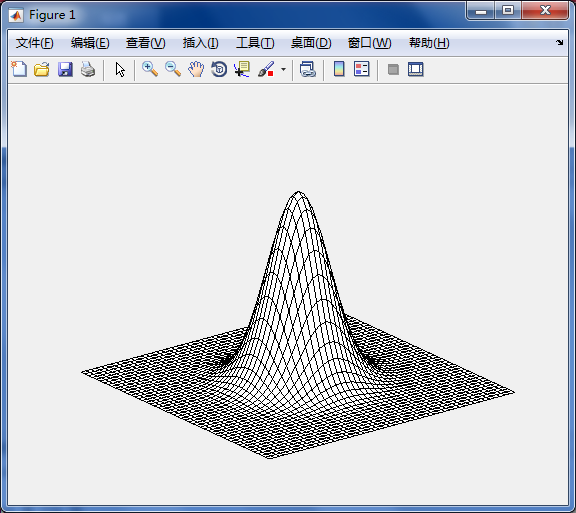H = fftshift(lpfilter('gaussian',500,500,50));
mesh(double(H(1:10:500,1:10:500)))
axis tight           %设置坐标轴显示范围为紧凑型
colormap([0 0 0])
axis off
grid off
view(-25,0)        %将观察者的视角设置为方位角为-25且仰角设置为0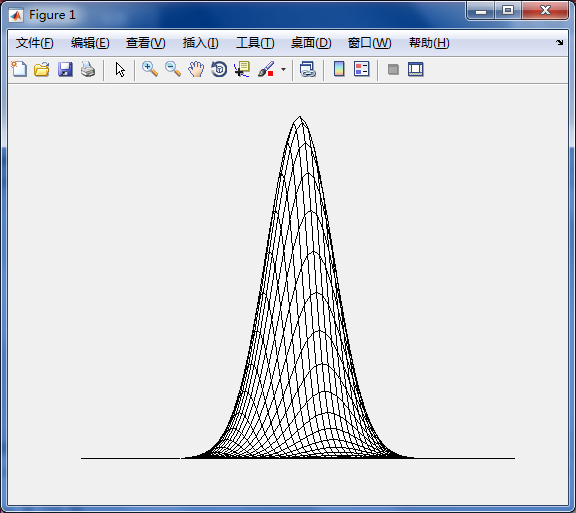view(-10,-10)      %将观察者的视角设置为方位角为-10且仰角设置为-10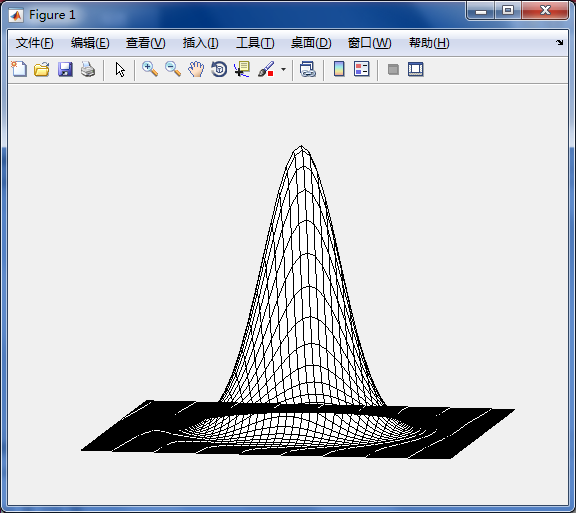surf(H)


H = fftshift(lpfilter('gaussian',500,500,50));
surf(double(H(1:10:500,1:10:500)));
axis tight
colormap(gray)           %将颜色转为灰度
[Y,X] = meshgrid(-2:0.1:2,-2:0.1:2);   %用于产生坐标值
Z = X.*exp(-X.^2 - Y.^2);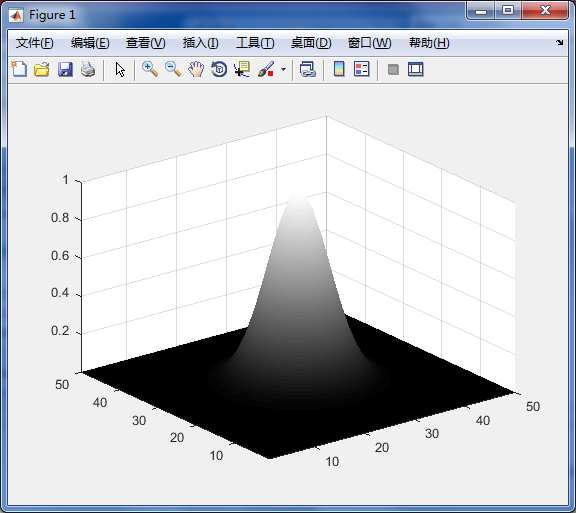# （二）高通（锐化）频域滤波器

function H = hpfilter(type, M, N, D0, n)
if nargin == 4
n = 1; %默认值为1
end
Hlp = lpfilter(type,M,N,D0,n);
H = 1-Hlp;


H1 = fftshift(hpfilter('ideal',500,500,50));
H2 = fftshift(hpfilter('gaussian',500,500,50));
H3 = fftshift(hpfilter('btw',500,500,50));
mesh(double(H1(1:10:500,1:10:500)));
mesh(double(H2(1:10:500,1:10:500)));
mesh(double(H3(1:10:500,1:10:500)));
axis tight
axis off
subplot(131),imshow(H1), title('理想高通滤波器');
subplot(132),imshow(H2), title('高斯高通滤波器');
subplot(133),imshow(H3), title('巴特沃斯高通滤波器');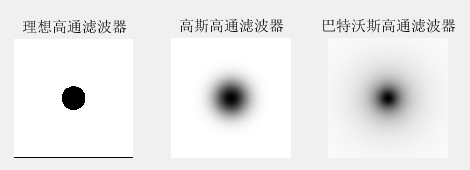f = imread('C:\Users\Public\Pictures\Sample Pictures\flower.jpg ');
D0 = 0.05*PQ(1);
H = hpfilter('ideal',PQ(1),PQ(2),D0);
g = dftfilt(f,H);
subplot(121),imshow(f),title('原始图像');
subplot(122),imshow(g,[]),title('理想高通滤波器后的图像');


（图一）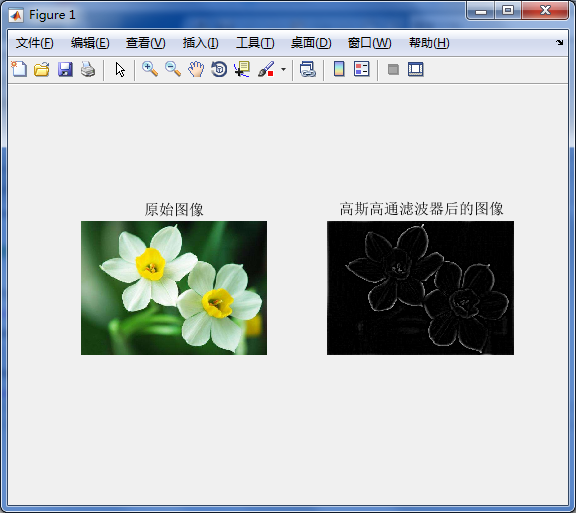（图二）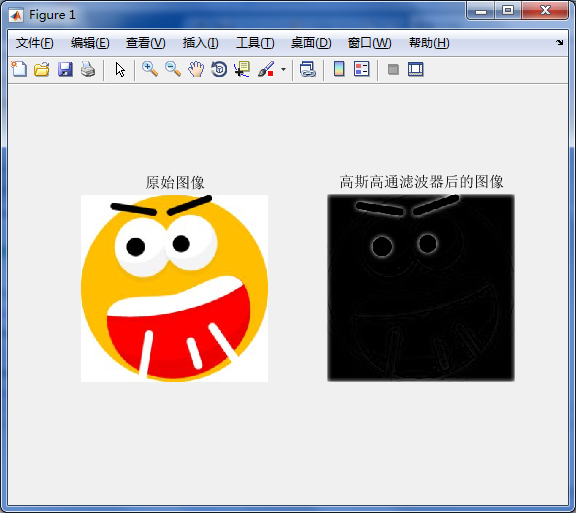f = imread('C:\Users\Public\Pictures\Sample Pictures\flower.jpg ');
D0 = 0.05*PQ(1);
H = hpfilter('btw',PQ(1),PQ(2),D0);
g = dftfilt(f,H);
subplot(121),imshow(f),title('原始图像');
subplot(122),imshow(g,[]),title('巴特沃斯高通滤波器后的图像');


（图三）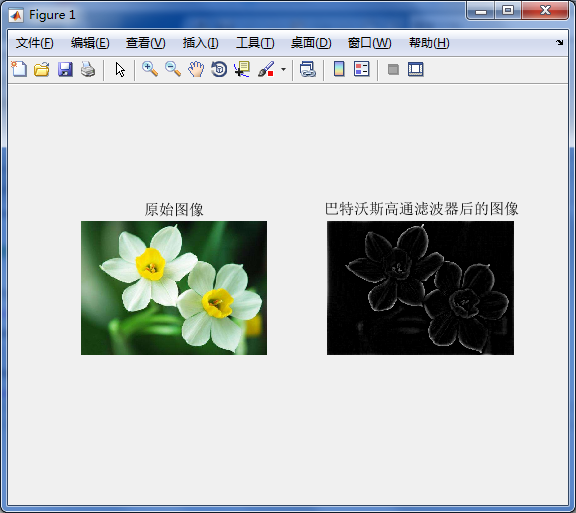>> f = imread('C:\Users\Public\Pictures\Sample Pictures\timg.jpg ');
D0 = 0.05*PQ(1);
H = hpfilter('ideal',PQ(1),PQ(2),D0);
g = dftfilt(f,H);
subplot(121),imshow(f),title('原始图像');
subplot(122),imshow(g,[]),title('理想高通滤波器后的图像');


（图四）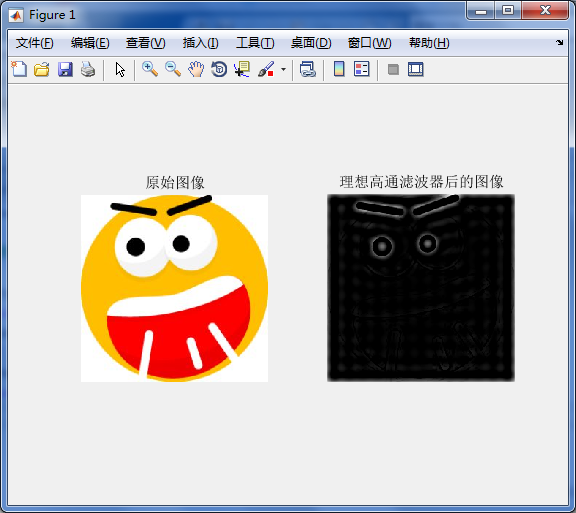# （三）选择性滤波

## （1）带通和带阻滤波器

function H = bandfilter(type,band,M,N,D0,W,n)
if nargin < 7
n = 1;  %默认值
end
switch lower(type)
case 'ideal'
H = idealReject(D,D0,W);
case 'btw'
H = btwReject(D,D0,W,n);
case 'gaussian'
H = gaussianReject(D,D0,W);
otherwise
error('Unknown filter type.')
end

if strcmp(band,'pass')
H = 1 - h;
end

%------------------------------------------------------------------%
function H = idealReject(D,D0,W)
RI = D <= D0 - (W/2);
RO = D >= D0 + (W/2);
H = tofloat(RO|RI);
%------------------------------------------------------------------%
function H = btwReject(D,D0,W,n)
H = 1./(1 + ((D*W)./(D.^2 - D0^2)).^2*n));
%------------------------------------------------------------------%
function H = gaussianReject(D,D0,W)
H = 1 - exp(-((D.^2 - D0^2)./(D.*W + eps)).^2);


## （2）陷波带阻和陷波带通滤波器

f = imread('C:\Users\Public\Pictures\Sample Pictures\flower.jpg ');
f=rgb2gray(f);
[M,N] = size(f);
[f,revertclass] = tofloat(f);
F = fft2(f);
S = fftshift(log(1+abs(F)));    %将直流分量移到频谱中心
S = gscale(S);   %频谱
subplot(221),imshow(f),title('原图像');
subplot(222),imshow(S),title('显示频谱图像');
C1 = [99 154;128 163];         %交互式的尖峰
H1 = cnotch('gaussian', 'reject',M,N,C1,5);      %陷波滤波器
P1 = gscale(fftshift(H1).*(tofloat(S)));         %计算滤波器变换的频谱
subplot(223),imshow(P1), title('滤波变换的频谱图像');
g1 = dftfilt(f,H1);       %滤波图像
g1=revertclass(g1);
subplot(224),imshow(g1), title('滤波图像');


function g=gscale(f,varargin)
if length(varargin)==0
method='full8';
else method=varargin{1};
end
if strcmp(class(f),'double')&(max(f(:))>1 | min(f(:))<0)
f=mat2gray(f);
end

switch method
case 'full8'
g=im2uint8(mat2gray(double(f)));
case 'full16'
g=im2uint16(mat2gray(double(f)));
case 'minmax'
low = varargin{2};high = varargin{3};
if low>1 | low<0 |high>1 | high<0
error('Parameters low and high must be in the range [0,1]')
end
if strcmp(class(f),'double')
low_in=min(f(:));
high_in=max(f(:));
elseif  strcmp(class(f),'uint8')
low_in=double(min(f(:)))./255;
high_in=double(max(f(:)))./255;
elseif   strcmp(class(f),'uint16')
low_in=double(min(f(:)))./65535;
high_in=double(max(f(:)))./65535;
end

otherwise
error('Unknown method')
end


（图五）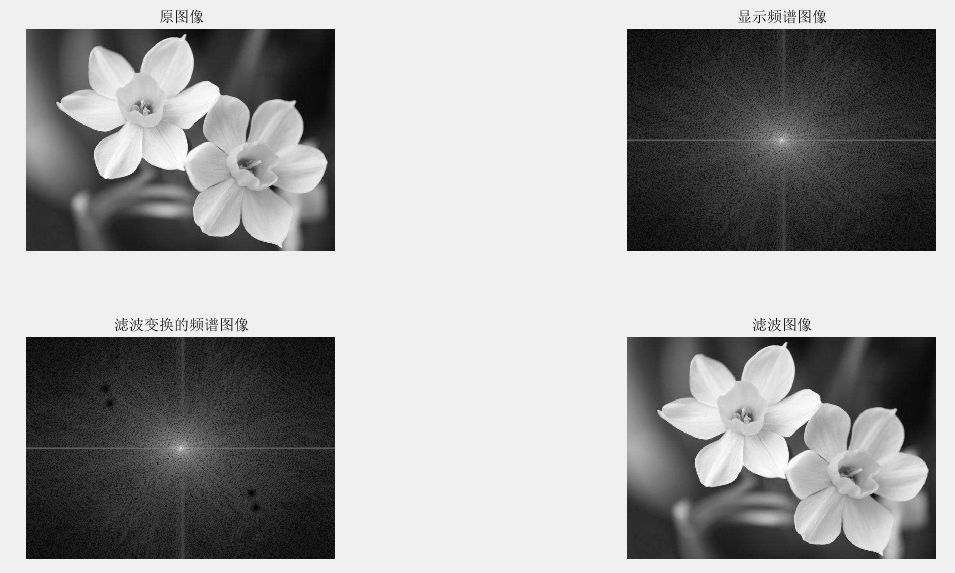（图六）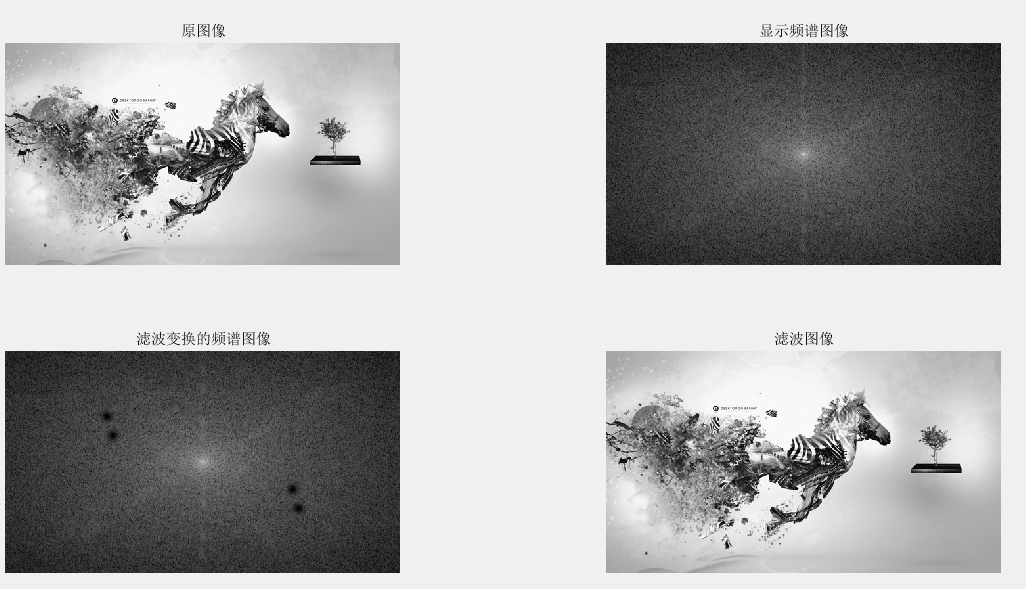2019-11-18 16:46:03 donaldsy 阅读数 9
• ###### MATLAB图像处理

全面系统的学习MATLAB在图像处理中的应用

19926 人正在学习 去看看 魏伟

## 相关理论

• 几何矩 $M_{j i}=\sum_{x, y}\left(P(x, y) \cdot x^{j} \cdot y^{i}\right)$，其中(i+j)和等于几就叫做几阶距

• 中心距$m u_{j i}=\sum_{x, y}\left(P(x, y) \cdot(x-\bar{x})^{j} \cdot(y-\bar{y})^{i}\right)$，其中$\bar{x}, \bar{y}$表示它的中心质点。

• 中心归一化距$\mathrm{nu}_{j i}=\frac{\mathrm{mu}_{j i}}{\mathrm{m}_{00}^{(i+j) / 2+1}}$

• 矩的概念介绍

• 图像中心Center(x0,y0)
$\mathrm{x}_{0}=\frac{m_{10}}{m_{00}} y_{0}=\frac{m_{01}}{m_{00}}$
• API介绍与使用-计算矩cv::moments

moments(
InputArray  array,//输入数据
bool   binaryImage=false // 是否为二值图像
)

contourArea(
InputArray  contour,//输入轮廓数据
bool   oriented// 默认false、返回绝对值
)

arcLength(
InputArray  curve,//输入曲线数据
bool   closed// 是否是封闭曲线
)


## 代码 & 效果展示

• 演示代码 - 步骤
• 提取图像边缘
• 发现轮廓
• 计算每个轮廓对象的矩
• 计算每个对象的中心、弧长、面积

#include <iostream>
#include <opencv2/opencv.hpp>
#include <opencv2/imgproc/types_c.h>

using namespace std;
using namespace cv;

#ifndef P31
#define P31 31
#endif

#if P31 //图形矩形
Mat src, gray_src;
int threshold_value = 80;
int threshold_max = 255;
const char* output_win = "image moents demo";
RNG rng(12345);

void Demo_Moments(int, void*) {
Mat canny_output;
vector<vector<Point>> contours;
vector<Vec4i> hierachy;

Canny(gray_src, canny_output, threshold_value, threshold_value * 2, 3, false);
findContours(canny_output, contours, hierachy, RETR_TREE, CHAIN_APPROX_SIMPLE, Point(0, 0));

vector<Moments> contours_moments(contours.size());
vector<Point2f> ccs(contours.size());
for (size_t i = 0; i < contours.size(); i++) {
contours_moments[i] = moments(contours[i]);
ccs[i] = Point(static_cast<float>(contours_moments[i].m10 / contours_moments[i].m00), static_cast<float>(contours_moments[i].m01 / contours_moments[i].m00));
}

Mat drawImg;// = Mat::zeros(src.size(), CV_8UC3);
src.copyTo(drawImg);
for (size_t i = 0; i < contours.size(); i++) {
if (contours[i].size() < 100) {
continue;
}
Scalar color = Scalar(rng.uniform(0, 255), rng.uniform(0, 255), rng.uniform(0, 255));
printf("center point x : %.2f y : %.2f\n", ccs[i].x, ccs[i].y);
printf("contours %d area : %.2f   arc length : %.2f\n", i, contourArea(contours[i]), arcLength(contours[i], true));
drawContours(drawImg, contours, i, color, 2, 8, hierachy, 0, Point(0, 0));
circle(drawImg, ccs[i], 2, color,2, 8);
}

imshow(output_win, drawImg);
return;
}
#endif

int main() {
std::string path = "../circle.JPG";

string str_input = "input image";
string str_output = "output image";

if (img.empty()) {
std::cout << "open file failed" << std::endl;
return -1;
}

namedWindow(str_input, WINDOW_AUTOSIZE);
namedWindow(str_output, WINDOW_AUTOSIZE);
imshow(str_input, img);

#if P31
src = img;
cvtColor(src, gray_src, CV_BGR2GRAY);
GaussianBlur(gray_src, gray_src, Size(3, 3), 0, 0);

char input_win[] = "input image";
namedWindow(input_win, WINDOW_AUTOSIZE);
namedWindow(output_win, WINDOW_AUTOSIZE);
imshow(input_win, src);

createTrackbar("Threshold Value : ", output_win, &threshold_value, threshold_max, Demo_Moments);
Demo_Moments(0, 0);
#endif

cv::waitKey(0);
cv::destroyAllWindows();
return 0;
}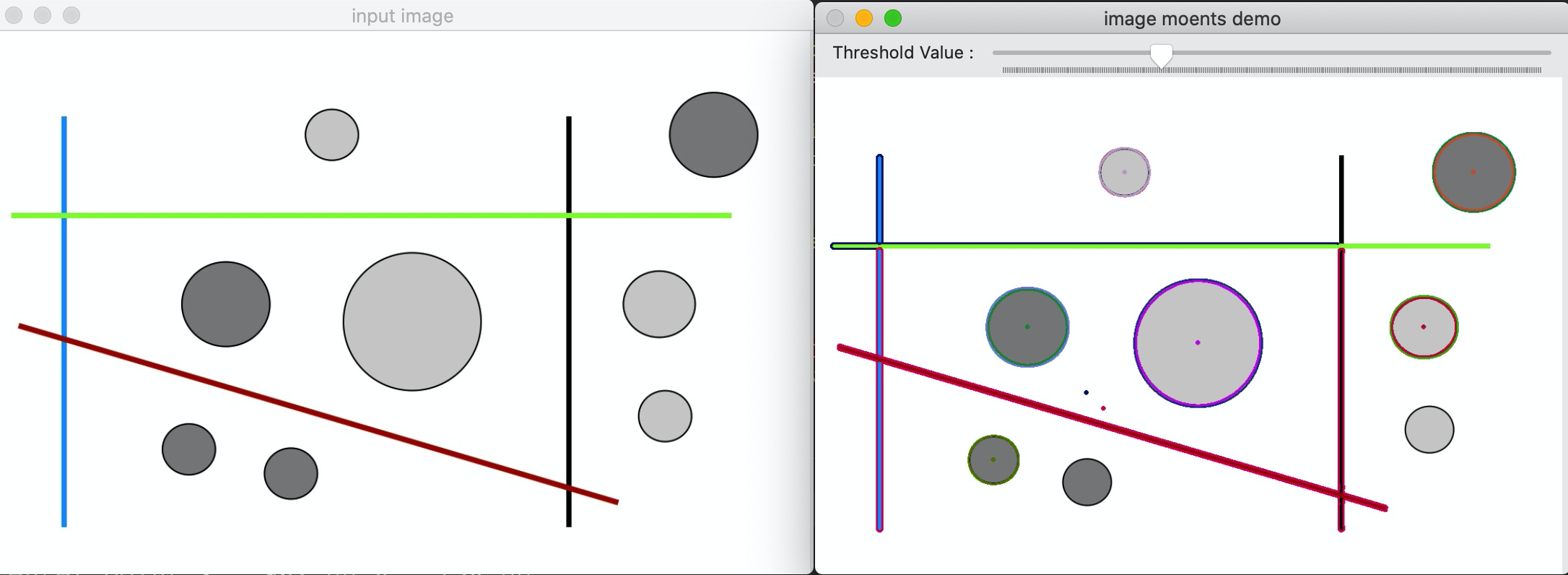2016-06-20 17:20:03 u010369450 阅读数 2153
• ###### MATLAB图像处理

全面系统的学习MATLAB在图像处理中的应用

19926 人正在学习 去看看 魏伟# 不变矩

## 简述不变矩及无偏估计与有偏估计

E(A'）= A

### 矩的定义p+q阶原点矩：p+q阶中心矩:### Hu矩

Hu于1961年首先提出了基于直角坐标系的规则矩的概念(来自文章Pattern Recognition by Moment Invariants)，之后运用规则矩的非线性组合得到了一套具备尺度不变性，平移不变性以及旋转不变性的矩不变量(来自文章Visual Pattern Recognition by Moment Invariant)。（本段还将增补）
Hu矩的定义，基本性质具备平移，旋转缩放不变性的7个不变矩表达式
7个表达式太长不再赘述，这里附上原文链接：

1，输入预处理后的图像。2，计算零阶以及一阶矩。3，计算三阶以下中心矩
4，归一化中心矩。 5，计算7个Hu不变矩### Zernike矩

Zernike矩为基于Zernike多项式的正交化函数，所利用的正交多项式集（完备性是指在数学及其相关领域中，当一个对象具有完备性，即它不需要添加任何其他元素，这个对象也可称为完备的或完全的。  正交的完备性在线性空间中就是指构成这个空间的基是相互正交的,即这个空间中所有的向量都可以由这组基线性表出,而且这些基又相互正交.）Zernike矩源自于Teague提出的正交矩思想，相较于Hu矩，其具备良好的旋转不变性，作为正交矩能够构造任意的高阶矩，运算为积分运算，因此对噪声不敏感。

Zernike矩是图像函数f(x,y)在正交多项式上的投影，其中正交多项式在单位圆内正交

Zernike矩提取方案为：

1，输入预处理后的图像。2，找出目标最小外接矩形。3，计算各阶矩
4，归一化Zernike矩。

### Zernike矩与Hu矩及其应用

1）优点：

2）缺点：

①不变矩对噪声很敏感，因此有其他作者提出相关不变矩对上述进行改进。

②矩计算时间比较长。

③矩是一种整体性质，如果物体的一部分被其他物体遮挡，则无法计算不变矩。比如说角点是一种描述局部的性质

④Hu提出的不变矩只能对区域进行检测，不能用于边界的检测；对于区域的检测，总体上来说，极半径不变矩与Hu矩的效果相当；但是Hu矩收到图形对称性的影响，而极半径不变矩不受到图形对称性的影响，所以对于对称图形来说，极半径不变矩的效果要优于Hu不变矩

3）应用：

Hu和Zernike矩都具备平移旋转以及缩放的不变性，但是各有特点。Hu的目标信息不是很全面，含有较多的冗余信息；Zernike矩是正交矩，能够构造出任意的高阶矩，包含信息全面，对噪声不敏感。可采用不变矩应用于人形识别算法。

# Harris角点检测

Harris角点检测由Chris Harris和Mike Stephens于1988年在A COMBINED CORNER AND EDGE DETECTOR一文中提出

## 角点检测基础

### 角点的定义以及发展：

#### 角点算法的发展：典型的角点检测算法有Harris算法以及CSS算法## Harris角点算法思想以及实现

### Harris角点性质：

Harris角点的实现：定义了灰度变化函数（窗函数*灰度平移前后变化差的平方）以及角点响应函数R